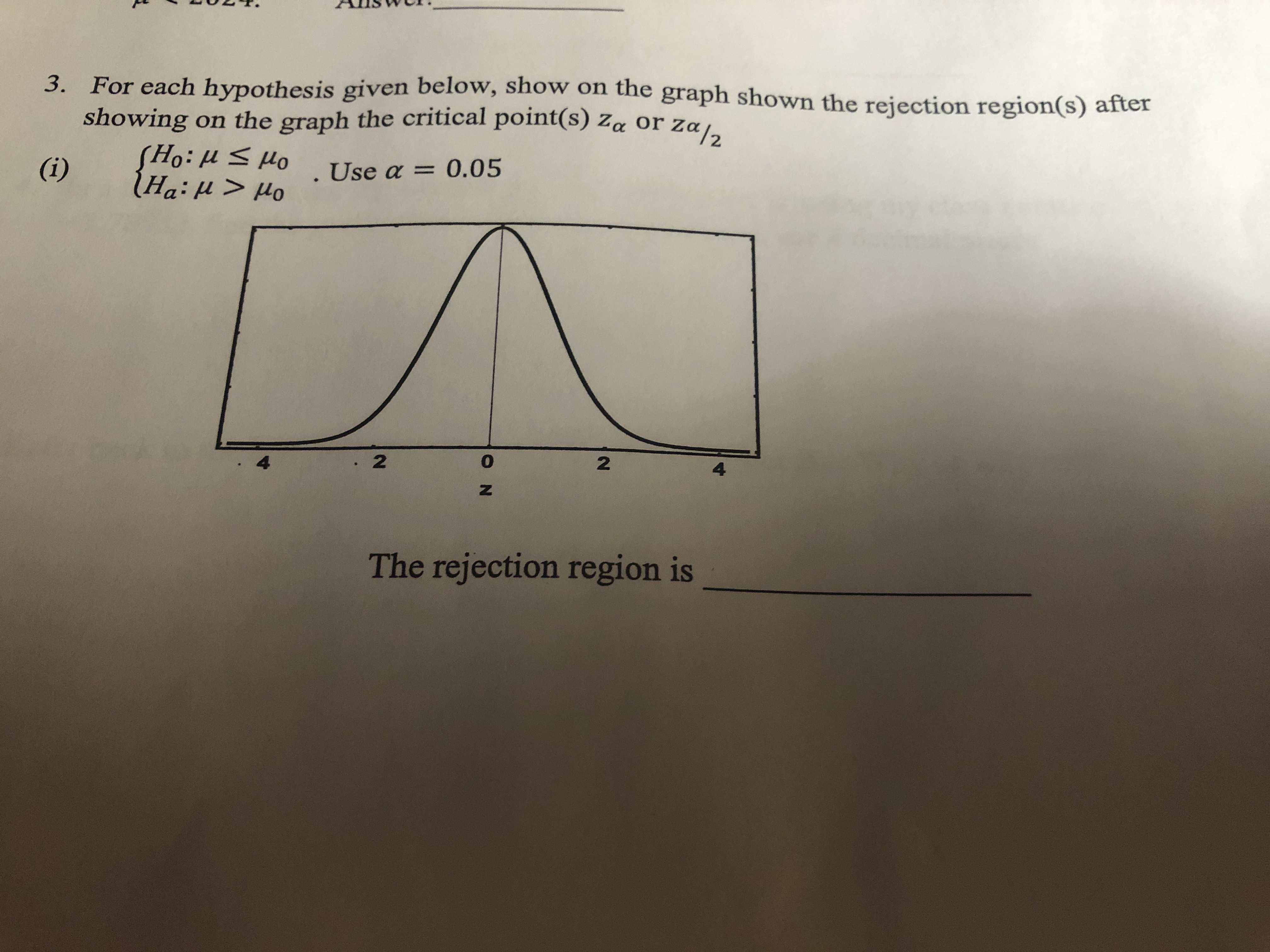# N ONT.Allswi3. For each hypothesis given below, show on the graph shown the rejection region(s) aftershowing on the graph the critical point(s) Za or Za/2(i)Ord 11:0SUse a 0.05424The rejection region is

Question
67 viewshelp_outlineImage TranscriptioncloseN O N T. Allswi 3. For each hypothesis given below, show on the graph shown the rejection region(s) after showing on the graph the critical point(s) Za or Za/2 (i) Ord 11:0S Use a 0.05 4 2 4 The rejection region is fullscreen
check_circle

Step 1

Introduction:

The given alternative hypothesis, Ha: μμ0 represents a right-tailed test.

Thus, the critical value would be = z0.05, such that PHo (Z) = PHo (Z0.05) = 0.05.

As a result, PHo (Z < ) = PHo (Z < z&...

### Want to see the full answer?

See Solution

#### Want to see this answer and more?

Solutions are written by subject experts who are available 24/7. Questions are typically answered within 1 hour.*

See Solution
*Response times may vary by subject and question.
Tagged in

### Hypothesis Testing